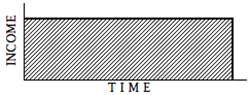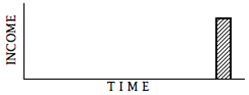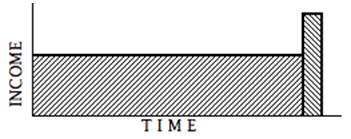# Lesson 18 – Property Reversion: Annuity plus Reversion Method (The Income Approach to Value)

Property Tax Rule 8, previously covered in Lesson 5, “Definition of the Income Approach and Property Tax Rule 8”, states, in subsection (b):
(b) Using the income approach, an appraiser values an income property by computing the present worth of a future income stream. … the income stream is divided into annual segments and the present worth of the total income stream is the algebraic sum (negative items subtracted from positive items) of the present worths of the several segments. In practical application, the stream is usually either (1) divided into longer segments, such as the estimated economic life of the improvements and all time thereafter or the estimated economic life of the improvements and the year in which the improvements are scrapped and the land is sold, …
….
In Lessons 16 and 17 we learned about the horizontally divided income described in subsection (b)(2). In this lesson we will learn about income streams divided vertically – in particular, a level terminal income stream combined with a single income payment sometime in the future – a reversion. You may sometimes hear the level terminal income stream called an annuity income, an annuity cash flow, or an Inwood annuity cash flow.

### Notes

1. William INWOOD (1771-1843) was an English architect and surveyor; he was the author of the Tables for the Purchasing of Estates, Freehold, Copyhold, or Leasehold, Annuities, Advowsons, &c., a forerunner of compound interest and annuity tables such as the AH 505.

#### Property Reversion: Annuity Plus Reversion Method

When the income stream is a series of equal, annual, incomes, that terminate in the future, the income stream is constant terminal shaped.When the income is a single payment some time in the future, the income is a reversion.Combine the two, and you have an annuity plus a reversion.Information needed:

• Net Income Before deducting for recapture and property Taxes [NIBT], or the requisite information to derive NIBT
• Value of Reversion
• Yield rate [Y]
• Effective Tax Rate [ETR]
• Time, such as Remaining Economic Life [REL], until the Reversion

After processing the market income stream to the Net Income Before deducting for recapture and property Taxes level [NIBT], the NIBT is capitalized into the value of the annuity income stream using the Capitalization Rate;  V = NIBT ÷ (YO + SFF{@Yo,Ann,REL} + ETR). The Reversion Income (Reversion Value) is the value attributable to the property remaining at the time of the property's reversion – this may be the end of the lease term, or perhaps the end of the property's Remaining Economic Life. Multiplying the Reversion Income by the reversion capitalization factor, which is comprised of the Yo plus the ETR, will give us the Value of the Reversion. Adding the Reversion Value to the Annuity Value will give us the Total Property Value. This can also be expressed in the following format:

Economic NIBT
(DIVIDE by)
Capitalization Rate ( YO + SFF{@Yo,Ann,REL} + ETR )
(equals)
Value of Level Terminal Income Stream
Future Value of Reversion
(TIMEs)
Reversion Factor PW1(@{Yo+ETR},Ann,REL)
(equals)
Value of Reversion
Value of Income Stream
(plus)
Value of Reversion
(equals)
Total Property Value
Where:
YO
Yield Rate for the overall property
SFF
The Sinking Fund Factor, at the Yield Rate, YO, with Annual compounding, for the remaining time until the Reversion, such as the Remaining Economic Life of the improvements or equipment.
REL
Remaining Economic Life – the remaining economic life of a property is the estimated period during which improvements will continue to contribute to the property value
ETR
Effective Tax Rate

#### EXAMPLE 18-1: Property Reversion Method

NIBT:
\$10,000
ETR:
1½%
reversion value:
\$100,000
REL:
10 years
to be received in
10 years
Y:
10%
NIBT:
\$10,000
(DIVIDE by)
Capitalization Rate:
÷ 0.177745
(10% Y + 0.062745 SFF{10%,Ann,10yr} + 1½% ETR)
(equals)
Value of Income =
\$56,260

FV of Reversion:
\$100,000
(TIMEs)
Reversion Factor:
× 0.336706
PW1{@(10% + 1½%),Ann,10yrs}
(equals)
PV of Reversion =
\$33,671

PV of Income Stream:
\$56,260
(plus)
PV of Reversion:
+ 33,671
(equals)
Total Property Value =
\$89,931

Here’s another example. In this scenario the appraiser has determined that the typical investor for this type of property will hold on to the investment for six years. The appraiser concludes that the value to this typical investor is how the market would value the property.

#### EXAMPLE 18–2: Valuation Using Inwood Annuity plus Reversion Method

Below are the assumptions on which the valuation is to be based. The income to be capitalized is estimated on a market basis and is processed to the level of Net operating Income Before deducting for recapture and ad valorem property Taxes [NIBT]. The reversionary value is estimated based on current value and anticipated inflation. A component for property taxes must be included in the capitalization rate.

Assumptions

Expected holding period [HP]
6
Years
Rentable area
65,000
Sq Ft
Annual rent (gross rent basis)
\$24.50
/ sq ft rentable area
Annual vacancy and collection loss
6%
of economic gross income
Ann. operating expenses (ex property taxes)
\$7.75
/ sq ft rentable area
Overall yield rate (YO)
12%
Ad valorem property tax rate
1%
Value of the property in 6 years
\$8,590,000

Estimated Annual Cash Flows

PGI:
\$1,592,500
less
V&CL:
− \$95,550
equals
Eff GI:
= \$1,496,950
less
Operating Expenses:
− \$503,750
equals
NIBT:
= \$933,200

Present Value Calculations

The capitalization rate is based on the 12% Yield rate plus the Sinking Fund Factor (for the six year Holding Period) plus the 1% Effective Tax Rate.

Yo
12%
SFF{@Y,Ann,HP}:
+ 0.123226
SFF{12%,Ann,6yrs}
ETR:
+ 1%
Capitalization Rate:
= 0.253226
Σ (Y + SFF + ETR)

The Reversion factor is based on the Yield rate plus the Effective Tax Rate (in this case, 12%+1%=13%), for the Holding Period, six years.

PW1{@(Y+ETR),Ann,HP}: 0.480319 PW1{13%,Ann,6yrs}

PV
Annual Income [NIBT]:
993,200 ÷ 0.253226 =
3,922,188
Reversion:
8,590,000 × 0.480319 =
+ 4,135,940
Total present value:
8,048,128
The estimated value of the property is, say:
\$8,050,000

### Summary

The lesson you just read covered the Property Reversion Capitalization, sometimes called the Inwood Annuity plus Reversion Method. The next lesson will address the valuation of personal property, and will use Property Reversion Capitalization.

Note: Before proceeding on to the next lesson, be sure to complete the exercises for this lesson.